# Kerala Plus One Chemistry Important Questions

Class 11 is a crucial year for most as this is when they get to choose subjects of their choice and opt for a stream of their preference on the basis of their future plans to go for higher studies in any particular stream. At the same time, the class 11 subjects give students a steady foundation for their class 12 and also for the competitive exams. Chemistry is especially important for students who wish to take up engineering or medical profession in the future. They need to prepare well for the boards and final class 11 exams also. For this, they can find help with Kerala Plus One Chemistry important questions.

These questions cover all the important topics of the subject, as per the Kerala Plus One Chemistry syllabus. This also gives students more practice in writing the exams and they are more confident of the upcoming exams. Students prepare well for the exams and are able to face it without much fear. See, here some of the important questions of Class 11 Chemistry Kerala state higher secondary board, which we have compiled here to help the students:

1.Predict if there will be any participation by mixing 20ml of 1.5 x 10⁻⁵ (M) BaCl₂ solution with 40ml of 0.9 x 10⁻⁵ (M) Na₂SO₄ solution (KsP of BaSO₄=1x 10¹º)

2. Calculate the entropy change involved in the vapourization of water at 373K to vapour at the same temperature (Latent heat of vaporization=2.275kJ/g)

3. Write reactions of extraction of silver by cyanide process

4. Alkaline hydrolysis of tertiary butyl bromide follows rate equation

Rate= K[ Alkyl (halide)]

1. Name the mechanism of the above reaction
2. Show the mechanism

5. (a) List the atoms with following electronic configuration in order of increasing ionisation energy

(i) He ] 2p⁶3s¹ (ii) He ] 2p⁵ (iii) He ] 2s² 2p⁶

(b) What is the type of hybridisation in each carbon atom in the compound H₃C-C_= CH

6. Write a balanced equation for the following:

(i) Potassium iodide is treated with acidified KMNO₄ solution

(ii) Potassium dichromate(acidified) reacts with hydrogen sulphide

(iii) Acidified Potassium dichromate reacts with oxalic acid

7. (a) Write balanced equations for the following named reactions:

(i) Friddel-Craft acylation

(ii) Corey-house reaction

(iii) Clemmensen reduction

(b) Identify ABCD:

(I)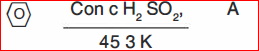(II)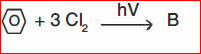(III)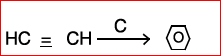(IV)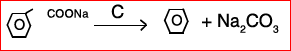8. (a) What is the molarity of a solution obtained by mixing 750ml of 0.5 M HCl with 250ml of 2(M) HCl?

(b) 2g of metal carbonate is neutralized completely by 100ml of 0.1 (N) HCl. What is the equivalent weight of metal carbonate?

9. 1.0 g of Mg is burnt in a closed vessel which contains 0.5g of O2. Which is the limiting reactant? What is the amount of MgO formed in the reaction?

2Mg + O₂2 MgO [Mg=24., O=16]

10. Give two reactions where hydrogen behaves as

(i) reducing agent (ii) oxidizing agent

11. (i) Write the bond line structure of the following compounds:

(a) 3, 4-dimethyl hept-2, 4 diene

(b) Cyclopentanol

(ii) Which alkaline has higher boiling point : n-hexane or isopentane and why?

12. Match the following :

(i) Nucleophiles (a) Unsaturated hydrocarbons.

(ii) Plaster of Paris (b) Electron deficient system.

(iii) Br2 + CCl4 (c) Lithium

(iv) Forms superoxides (d) Electron rich system.

(e) CaSO4 . ½ H2O

(f) Potassium

13. Balance the following redox reaction by ion-electron method:

KMnO4 + FeSO4 + H2SO4 > Fe2 (SO4)3 + MnSO4 +

K2SO4 + H2O (acidic medium)

14. (i) A 100% pure sample of a divalent metal carbonate weighing 2g on complete thermal decomposition releases. 448 cc. of CO2 at STP. What is the equivalent mass of the metal ?

(ii) Draw the electron dot diagram of HClO4.

15. Draw the molecular orbital diagram of O2. Find the bond order.

16. Write the electronic configurations of the following ions:

1. Ca²⁺ (Ca=20) (b) Br⁻ (Br= 35)

17. Write the IUPAC names of the following compounds: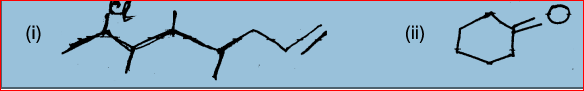18. (a) Why do sodium catch fire when dropped in water?

(b) Name the following:

(i) Alkaline earth metal which is least reactive

(ii) Alkaline earth metal which forms strongest base

(iii) Alkali metal is liquid at temperatures above 30 C

(iv) Alkali metal which is the strongest reducing agent

19. (a) Write the electronic configuration of the Cr atom (Cr=24). How many unpaired electrons are there? What is it’s magnetic behaviour?

(b) What will be the designation of the following orbitals?

i) n=3, l=0 , (ii) n=5, l=2

20. (i) Explain:

a) The relative strength of following acids is

Cl2CHOOH > ClCH2COOH>CH2COOH.

b) Methyl chloride is more reactive than chloro Benzene.

(ii) How will you prepare the following compounds :-

[Give reactions]

a) Ethane from acctaldehyde

b) Ethyne from iodoform (CHI3)

c) Ethene from ethanol.